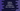# Python 3 program to check if a number is positive, negative or zero## Python 3 program to test if a number is positive or negative :

In this tutorial, we will learn how to test if a number is positive or negative. We will also check if the number is zero. This is a beginner-friendly python tutorial. With this example, you will learn how to read a user input, how to put your code in a different method to organize it, and how to use an_ if, else-if, else _condition. The program will take the number as input from the user, it will check if it is zero, greater than zero or less than zero and print out the result to the user. You can also store the number in a variable and check its value. But in this program, we are reading the number as an input from the user.

## Algorithm :

The algorithm of the program is like below :

1. Take the number as an input from the user. You can create one separate variable to hold the number or you can directly test the number. In this example, we are using one separate variable to hold it.
2. Check the number using one_ if-elseif-else_ condition. This condition will compare the number two times. The first one will check if it is equal to zero or not, the second one will check if it is greater than zero or not.

If both of these conditions fail, we will print that the number is less than zero or it is a negative number.

### Example Program :

``````def check_number(n):
if n == 0:
print ("Zero")
elif n > 0:
print (n,"is greater than zero")
else :
print (n,"is less than zero")

user_no = int(input("Enter a number : "))

check_number(user_no)``````

You can also download this program from here.

### Explanation :

1. check_number is a method to check if the number is zero, greater than zero or less than zero. This method takes one number as its argument. It doesn’t return anything.
2. Inside the method, we are using one if-elif-else condition. This condition will test the number and print out the result accordingly.
3. First, it will move inside the ‘if’ block. This block is used to check if the number is equal to zero or not. If the number is equal to zero, it will print one message “Zero” on the console and exit the if-elif-else block.
4. If the ‘if’ block fails, it will move into the ‘elif’ block. ‘elif’ is checking if the number is greater than zero or not. If it is greater than zero or if it is a positive number, it will print one message on the console and exit from the if-elif-else block.
5. If the ‘elif’ block fails, it will move to the last block. This is the ‘else’ block. Note that we are not verifying anything in this block. This block will run if the number is not equal to zero and if it is not greater than zero or this block will run only if the number is less than zero or if it is a negative number. We are sure about that. So, without checking any condition, just print to the user that the number is less than zero.
6. For reading the user input, the input() method is used. This method returns the value in string form. We are wrapping it with int() to get the integer value of the user input.